# Complex Numbers Chapter Exam

Exam Instructions:

Choose your answers to the questions and click 'Next' to see the next set of questions. You can skip questions if you would like and come back to them later with the yellow "Go To First Skipped Question" button. When you have completed the practice exam, a green submit button will appear. Click it to see your results. Good luck!

### Page 1

#### Question 1 1. How many roots does the equation below have?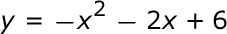#### Question 2 2. What type of solution(s) would you get from the equation below?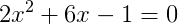#### Question 4 4. Which statement MUST be true about the equation below?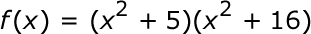### Page 2

#### Question 6 6. Which number is represented by the graph below?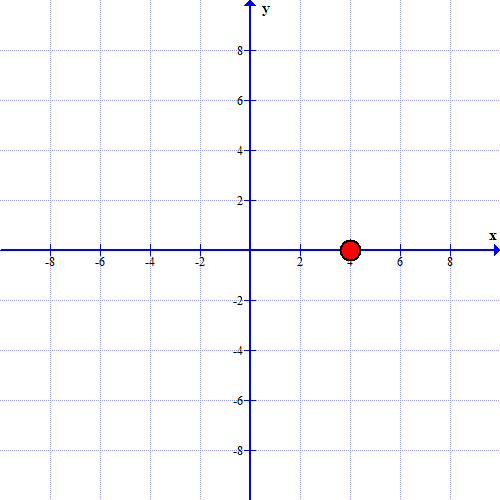#### Question 7 7. The graph below represents which complex number?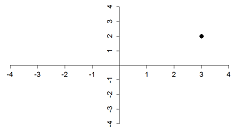#### Question 8 8. What is the complex conjugate of the expression below?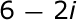### Page 3

#### Question 13 13. Simplify.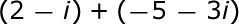#### Question 14 14. Solve the equation below: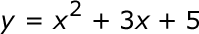### Page 4

#### Question 16 16. Simplify.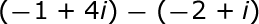#### Question 20 20. What is the most simplified form of the expression below?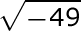### Page 5

#### Question 22 22. What is the simplified value of the expression below?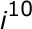#### Question 23 23. Simplify.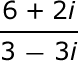#### Question 24 24. Find the roots of the equation below: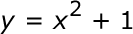### Page 6

#### Question 27 27. What would be the first step in dividing the complex numbers below?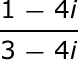#### Question 30 30. Simplify.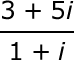#### Complex Numbers Chapter Exam Instructions

Choose your answers to the questions and click 'Next' to see the next set of questions. You can skip questions if you would like and come back to them later with the yellow "Go To First Skipped Question" button. When you have completed the practice exam, a green submit button will appear. Click it to see your results. Good luck!

Support